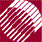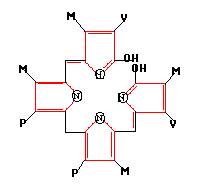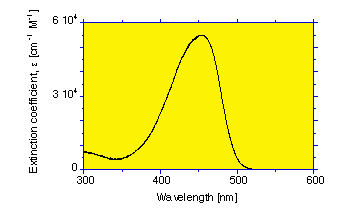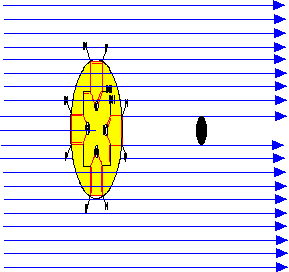ECE532 Biomedical Optics © 1998 Steven L. Jacques, Scott A. Prahl Oregon Graduate Institute

# Example: Bilirubin

The bilirubin molecule is a chromophore encountered when a newborn infant suffers from "jaundice", a syndrome in which the skin presents a yellow color. Bilirubin is a breakdown product of hemoglobin. Often there is significant hemolysis of red blood cells during child birth contributing to a transient bilirubin load. Normally, bilirubin binds to the serum protein albumin and is carried to the liver where enzymes convert it into a water-soluble form which is removed from the blood into the bile. But in newborns who have not yet developed sufficient enzymes to accomplish this task, the bilirubin accumulates in the blood, exceeds the holding capacity of the albumin, and spills into the skin to give the yellow skin color, and into the brain to cause irreversible brain damage (kernicterus). See article on optically monitoring bilirubinemia.

Let's approximate the values of A, ρa, µa, Qa, and σa for bilirubin.The structure of bilirubin is shown above. The diameter is approximately 1 nm. So the geometrical area is A = 4.5x10-15 cm2.

The extinction coefficient of bilirubin varies with wavelengthAt 460 nm, the extinction coefficient of bilirubin is ε = 53,846 [cm-1M-1] (see bilirubin spectrum).

The extinction coefficient ε [cm-1M-1] quoted in the literature is based on spectrometer measurements reported as T = 10-εCL where C is concentration [M] and L is pathlength [cm]. Therefore,

µa = εC ln(10)

A typical jaundiced neonate might have a bilirubin concentration of 10 mg/dL, or (0.100 g/liter)/(574.65 g/mole) = 0.17x10-3 M. In such a case, the bilirubin absorption coefficient at 460 nm is roughly

µa = εC ln(10) = (53846 [cm-1M-1])(0.17x10-3 M)(2.3) = 21 cm-1.The concentration C is equivalent to

ρa =

(0.17x10-3 [moles/liter])(6x1023 [mole-1])/(1000 cm3/liter] = 1.02x1017 [cm-3]

The efficiency of absorption is estimated:

Qa = µa/(ρaA) = (21 [cm-1])/((1.02x1017 [cm-3])(A = 4.5x10-15 [cm2])) = 0.046

The effective cross-section is σa = QaA = (0.046)(4.5x10-15 [cm2]) = 2.1x10-16 [cm2]. Bilirubin's effective cross-sectional diameter is sqrt(.046) or 21% the size of its geometrical diameter.

Keep in mind that the wavelength of blue light (460 nm) is about 460-fold greater than the diameter of the bilirubin chromophore. So collection of the electromagnetic wave of a photon by the "antenna" of bilirubin is analogous to a how small radio antenna collects the electromagnetic wave from a radio station (a 1000 MHz radio frequency -> 300 m wavelength). The concept of a "shadow" cast by an opaque chromophore is merely a memory device to remember the definitions.